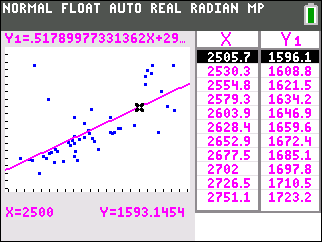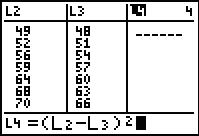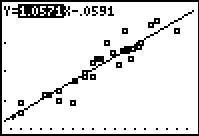### Does a Correlation Exist?

Students determine, by examining a graph, if a data set has a positive or negative correlation coefficient.
•TI-84 Plus CE
•TI-84 Plus C Silver Edition
• TI-84 Plus Silver Edition
• TI-84 Plus### Transforming Relationships

Students assess the strength of a linear relationship using a residual plot and calculate the correlation coefficient and coefficient of determination to assess the data set.
•TI-84 Plus CE
•TI-84 Plus C Silver Edition
• TI-84 Plus Silver Edition
• TI-84 Plus### Square it Up!

Students investigate the method of least squares by finding the residuals and the sum of the squares of the residuals.
•TI-84 Plus CE
•TI-84 Plus C Silver Edition
• TI-84 Plus Silver Edition
• TI-84 Plus